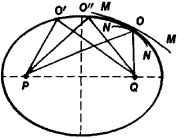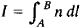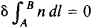# Fermat's Principle

Also found in: Dictionary, Wikipedia.
Related to Fermat's Principle: Snell's law, Huygens principle

## Fermat's principle

[fer′mäz ′prin·sə·pəl]
(optics)
The principle that an electromagnetic wave will take a path that involves the least travel time when propagating between two points. Also known as least-time principle; stationary time principle.

## Fermat’s Principle

a fundamental principle of geometrical optics. In its simplest form it consists in the assertion that a ray of light between two points in space always follows the path along which its travel time is less than along any of the other paths connecting the points. The time required by light to travel a distance I in a medium with a refractive index n is proportional to the optical path S. For a homogeneous medium, S = ln; for a variable n,

S = ∫ln dl

It may therefore be said that Fermat’s principle is the principle of the minimum optical path.

As originally formulated by P. Fermat around 1660, Fermat’s principle was taken as the most general law of the propagation of light; all the laws of geometrical optics then known followed from it. For a homogeneous medium the principle reduces to the law of the rectilinearity of a ray of light (in accordance with the axiom of geometry that a straight line is the shortest distance between two points). For the case of a ray incident on the interface between different media, the laws of the reflection and refraction of light can be obtained from Fermat’s principle.

In its more rigorous formulation Fermat’s principle is a variational principle asserting that a real ray of light from one point to another follows the path along which its travel time either is an extremum in comparison with the travel times along the other paths joining the points or is the same for all paths. In other words, the optical path of a ray may be not only less than but also greater than or equal to the other possible paths connecting the points. Examples of the minimum path are the above-mentioned propagation of light in a homogeneous medium and the transmission of light across the interface between two media with different refractive indices n.Figure 1. Fermat’s principle: the actual path of the light corresponds to an extremum of the propagation time

The three cases (the path of a ray is a minimum, a maximum, or equal to the other possible paths) can be illustrated by analyzing the reflection of a ray from a concave mirror (Figure 1). Suppose the mirror has the form of an ellipsoid of revolution, and the light propagates from one focus P to the other Q (a path without reflection is impossible). It then follows from the properties of an ellipsoid that the optical path of the ray PO′ + O′Q is equal to the other possible paths, such as PO″ + O″Q. If on the path between P and Q the light is reflected from a mirror of smaller curvature (mirror MM) than that of the ellipsoid, the minimum path is realized. If, however, the light is reflected from a mirror of greater curvature (mirror NN), the maximum path is realized. The condition that the optical path be an extremum reduces to the requirement that the variation δI of the integral(where A and B are the points between which the light is propagated) must be equal to zero. Thus, the mathematical statement of Fermat’s principle, in the notation of the calculus of variations, is as follows:In the wave theory of light Fermat’s principle is the limiting case of the Huygens-Fresnel principle and is applicable when the diffraction of light may be ignored (when the wavelength of the light wave is sufficiently small in comparison with the characteristic dimensions of the problem). By regarding the rays as normals to the wave surfaces, it can easily be shown that for any propagation of light the optical paths will be extrema. In all cases where diffraction must be taken into account, Fermat’s principle ceases to be applicable.

### REFERENCES

Fermat, P. de. Oeuvres, vols. 1–4. Paris, 1891–1912.
Landsberg, G. S. Optika, 5th ed. (Obshchii kurs fiziki.) Moscow, 1976.
Crawford, F. Volny. (Berkleevskii kurs fiziki, vol. 3.) Moscow, 1974.
Born, M., and E. Wolf. Osnovy optiki, 2nd ed. Moscow, 1973. (Translated from English.)

A. P. GAGARIN

References in periodicals archive ?
Mustapha, "Light Deflection, Lensing, and Time Delays from Gravitational Poten-tials and Fermat's Principle in the Presence of a Cosmological Constant", Phys.
Here we implement Fermat's principle by constructing all possible propagation paths from the antenna to the boundary points, and then from the boundary points to the current point in p.
Snell's law follows from Fermat's principle of least time, which in turn follows from the propagation of light as waves.

Site: Follow: Share:
Open / Close